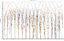# Difference between revisions of "TDLT"

This page holds the results of Time Domain Lapped Transform (TDLT) optimization problems looking for integer transform coefficients that provide optimal coding gain. Wherever possible the assumptions are stated. Later we should include testing against actual image data to verify the results (see test data here).

The coding gain objective used as the objective is taken from slide 13 of Tim's presentation An Introduction to Video Coding

<need figure with block matrix diagrams>

The free parameters are initially just the coefficients p_0,...,p_m,q_0,...,q_m where m=(n/2)-1. We limit these to being dyadic rationals, e.g., x/2^d with d=6, between [-1,1].

Given p's and q's and assuming a linear ramp constrains the s's.

## 4x8

Optimal real-valued coefficients for V:

p0 = -0.18117338915051454

q0 = 0.6331818230771687

CG = 8.60603

p0 q0 s0 s1 CG SBA Filterbank
R=f -11/64
-0.171875
36/64
0.5625
91/64
1.421875
85/64
1.328125

8.63473

22.0331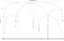R=t,D=f -12/64
-0.1875
41/64
0.640625
92/64
1.4375
1093/768
1.423177

8.60486

20.0573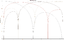R=t,D=t
max CG
-16/64
-0.25
41/64
0.640625
92/64
1.4375
93/64
1.453125

8.59886

18.9411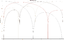R=t,D=t
max SBA
-8/64
-0.125
30/64
0.46875
136/64
2.125
91/64
1.421875

8.23230

25.1934
64px

## 8x16

Optimal real-valued coefficients for V:

p0 = -0.39460731547057293

p1 = -0.33002212811740816

p2 = -0.12391270981321137

q0 = 0.822154737511288

q1 = 0.632488694485779

q2 = 0.40214668677553894

CG = 9.56867

p0 p1 p2 q0 q1 q2 s0 s1 s2 s3 CG Filterbank
R=f -23/64
-0.359375
-18/64
-0.28125
-6/64
-0.09375
48/64
0.75
34/64
0.53125
20/64
0.3125
90/64
1.40625
73/64
1.140625
72/64
1.125
75/64
1.171875

9.60021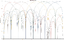R=t,D=f -26/64
-0.40625
-22/64
-0.34375
-8/64
-0.125
53/64
0.828125
41/64
0.640625
26/64
0.40625
11/8
1.375
879/768
1.14453125
1469/1280
1.14765625
275/224
1.2276785714285714

9.56627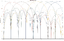R=t,D=t -24/64
-0.375
-20/64
-0.3125
-4/64
-0.0625
53/64
0.828125
40/64
0.625
24/64
0.375
88/64
1.375
75/64
1.171875
76/64
1.1875
76/64
1.1875

9.56161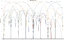## 16x32

Best-known real-valued coefficients for V (R=t):

p0 = -0.42111473798940136

p1 = -0.4121736499899753

p2 = -0.3350240707669929

p3 = -0.3224547931861314

p4 = -0.25883387978005545

p5 = -0.20951913473498104

p6 = -0.0598657149803332

q0 = 0.9107782439906195

q1 = 0.8109855829278226

q2 = 0.715846584586721

q3 = 0.6135951570714172

q4 = 0.49846644853347627

q5 = 0.3945215834922529

q6 = 0.21822275136248082

CG = 9.81157

p0 p1 p2 p3 p4 p5 p6 q0 q1 q2 q3 q4 q5 q6 s0 s1 s2 s3 s4 s5 s6 s7 CG Filterbank
R=f -24/64
-0.375
-23/64
-0.359375
-17/64
-0.265625
-12/64
-0.1875
-14/64
-0.21875
-13/64
-0.203125
-7/64
-0.125
50/64
0.78125
40/64
0.625
31/64
0.484375
22/64
0.34375
18/64
0.28125
16/64
0.25
11/64
0.171875
90/64
1.40625
74/64
1.15625
73/64
1.140625
71/64
1.109375
67/64
1.046875
67/64
1.046875
67/64
1.046875
72/64
1.125

9.89338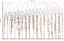R=t,D=f -26/64
-0.40625
-27/64
-0.421875
-22/64
-0.34375
-18/64
-0.28125
-16/64
-0.25
-14/64
-0.21875
-5/64
-0.078125
58/64
0.90625
52/64
0.8125
45/64
0.703125
36/64
0.5625
31/64
0.484375
23/64
0.359375
13/64
0.203125
3/2
1.5
77/64
1.203125
77/64
1.203125
1105/896
1.23326
218/192
1.135417
197/176
1.119318
1919/1664
1.153245
4351/3840
1.133073

9.79398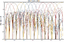R=t,D=t -16/64
-0.25
-16/64
-0.25
-8/64
-0.125
-17/64
-0.265625
-27/64
-0.421875
-13/64
-0.203125
-2/64
-0.03125
57/64
0.890625
50/64
0.78125
40/64
0.625
32/64
0.5
32/64
0.5
28/64
0.4375
12/64
0.1875
112/64
1.75
84/64
1.3125
88/64
1.375
80/64
1.25
72/64
1.125
72/64
1.125
73/64
1.140625
70/64
1.09375

9.65884